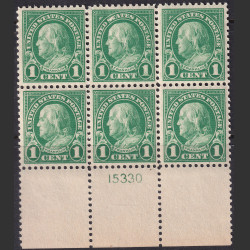Mint NH XF \$35.00 Plate number block of 6 (CV \$55)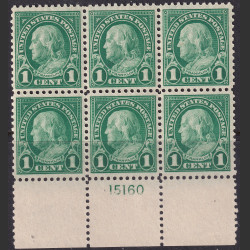Mint NH VF \$35.00 Plate number of 6 (CV \$55)Mint NH XF \$35.00 Plate number block of 6 (CV \$55)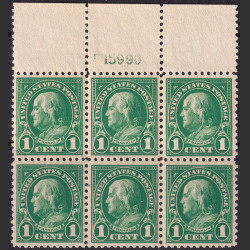Mint NH XF \$30.00 Plate number block of 6 (CV \$55)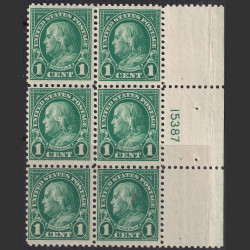Mint NH F-VF \$20.00 Plate number block of 6 (CV \$55)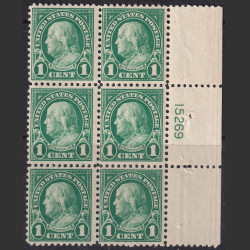Mint NH F-VF \$20.00 Plate number block of 6 (CV \$55)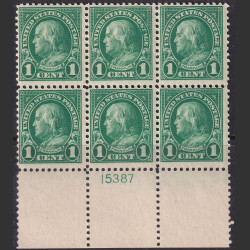Mint NH F-VF \$20.00 Plate number of 6 (CV \$55)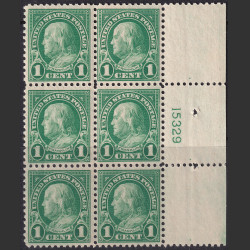Mint NH F-VF \$20.00 Plate number block of 6 (CV \$55)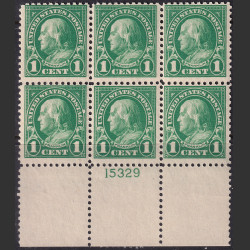Mint NH F-VF \$20.00 Plate number block of 6 (CV \$55)